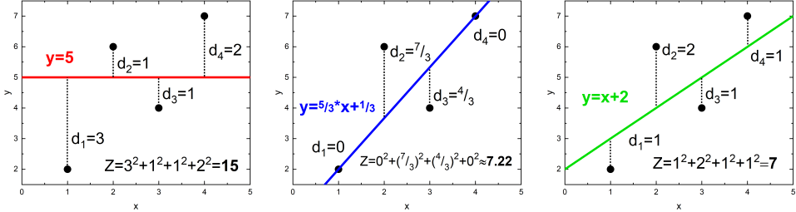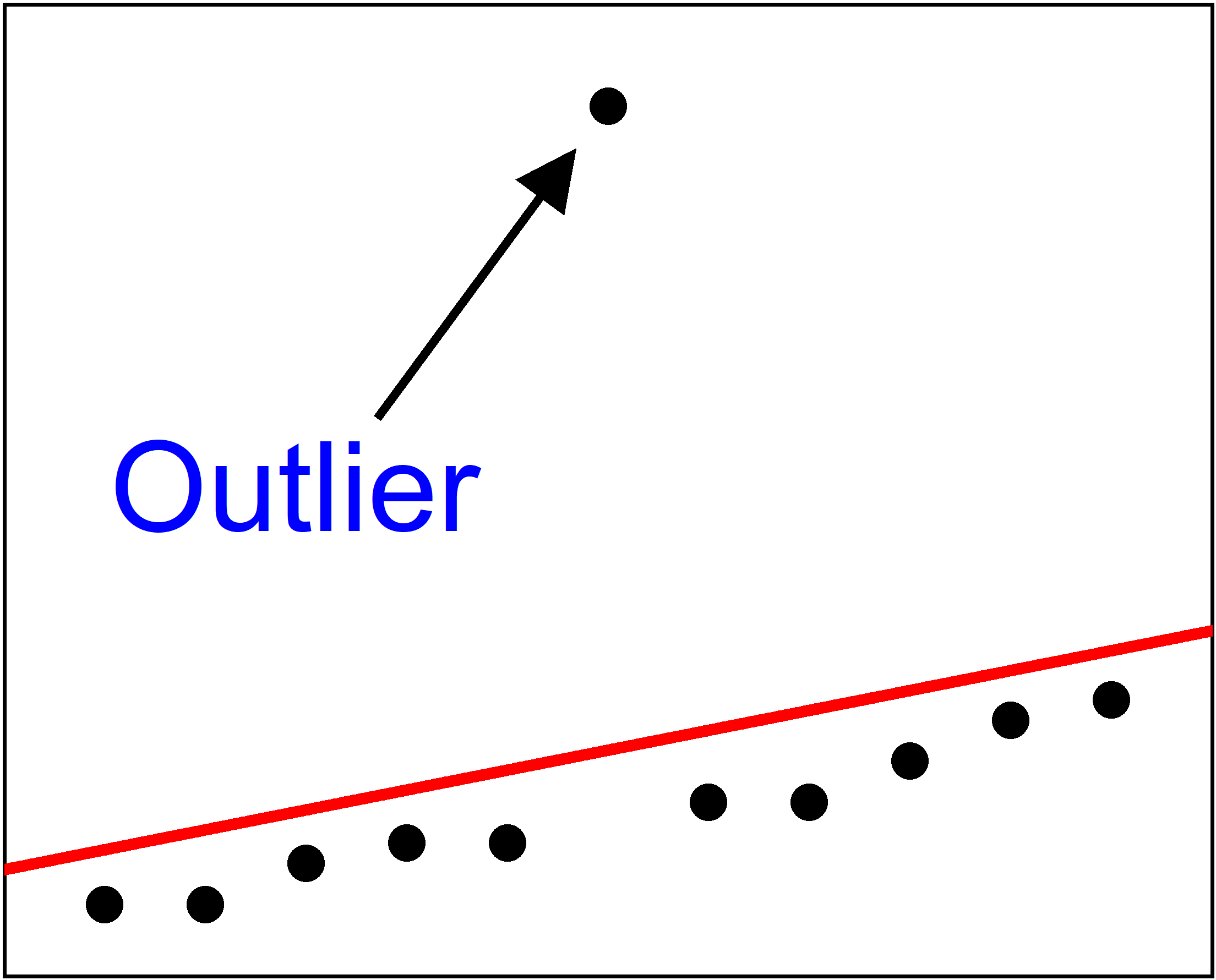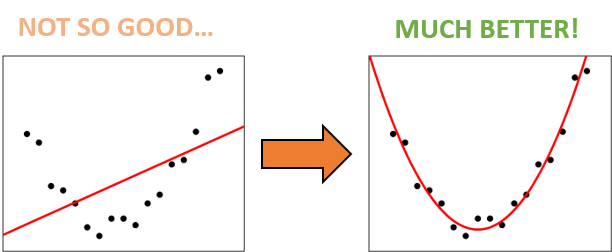# Least Squares Regression Line Calculator

Created by Wojciech Sas, PhD candidate
Reviewed by Bogna Szyk and Jack Bowater
Last updated: Dec 03, 2021

This is the least square regression line calculator - a user-friendly tool that answers the question "How to find the line of best fit?". If you are wondering how to find the average rate of change for a car that is increasing its velocity, then you are in the right place! In the article you can also find some useful pieces of information about the least square method, how to find the least squares regression line, and also what to pay particular attention to while performing a least square fit.

## How to find the line of best fit?

Intuitively, you can try to draw a line that passes as near to all the points as possible. Sometimes, it can be a straight line, which means that we will perform a linear regression. There are multiple methods of dealing with this task, with the most popular and widely used being the least squares estimation. Here we have some real-life examples:

The faster you drive, the more combustion there is in your car's engine. Maybe the winter is freezing cold, or the summer is sweltering hot, so you need to buy more electricity to use on heating on air conditioning. You can imagine many more similar situations where an increase in A causes the growth (or decay) of B.

Why do we use it? Well, with just a few data points, we can roughly predict the result of a future event. This is why it is beneficial to know how to find the line of best fit. It'll help you find what the ratio of B and A is at a certain time. Unlike the ratio calculator, which can deal only with one pair of numbers at once, this least squares regression line calculator shows you how to find the least square regression line for multiple data points.

## Least squares regression line equation

To make everything as clear as possible - we are going to find a straight line with a slope, a, and intercept, b. The formula for the line of the best fit with least squares estimation is then:

y = a * x + b.

As you can see, the least square regression line equation is no different that the standard expression for linear dependency. The magic lies in the way of working out the parameters a and b.

Great! So what does the least squares really mean? Jump to the next section to find out!

## Least squares method

Do you wonder how to find the line of best fit using the least square method? The idea is simple:1. Draw a straight line: f(x) = a*x + b
2. Evaluate all of the the vertical distances, dᵢ, between the points and your line: dᵢ = |yᵢ - f(xᵢ)|
3. Square them: dᵢ²
4. Sum them together, Z = ∑dᵢ² = d₁² + d₂² + d₃² + …
5. Find a line such that the value of Z becomes as little as possible.
6. Enjoy knowing the origin of the name of the least squares method.

It might sound a bit vague at first glance, so to make things a bit clearer, let's take a look at some pictures. There are three different lines fitted for the same data points, (1,2), (2,6), (3,4), (4,7):As you can see, Z has different values in each case. It's minimal for the third plot, but can we do even better? Use our least squares regression line calculator to find out if that's the most optimal solution!

## How to find the least squares regression line?

Or, in other words, how does our least squares regression line calculator work? We want to estimate the regression line parameters a and b. In the standard least square method, we can work out a few auxiliary values which will simplify the final formula:

• Sx = ∑xᵢ = x₁ + x₂ + x₃ + …
• Sy = ∑yᵢ = y₁ + y₂ + y₃ + …
• Sxx = ∑xᵢ² = x₁² + x₂² + x₃² + …
• Syy = ∑yᵢ² = y₁² + y₂² + y₃² + …
• Sxy = ∑xᵢyᵢ = x₁y₁ + x₂y₂ + x₃y₃ + …
• Δ = n*Sxx - Sx²

where n is the total number of points. The least square fit emerges from these coefficients:

• a = (n*Sxy - Sx*Sy) / Δ
• b = (Sxx*Sy - Sx*Sxy) / Δ

By solving these formulas, you receive some numerical values. But is there a way to decide how many significant digits should we include? Estimating the error of these parameters (in this case the standard deviations) will be handy:

• σa = √(n/(n-2) * (Syy - a * Sxy - b * Sy) / Δ)
• σb = √(Sxx / n) * σa

Now, look at the two significant digits from the standard deviations and round the parameters to the corresponding numbers of decimals. Remember to use scientific notation for really big, or really small, values.

In the end, we can also find the Pearson correlation coefficient, r:

• r = (n * Sxy - Sx * Sy) / √((n*Sxx - Sx²) * (n * Syy - Sy²))

The absolute value of r can span from 0 to 1. The closer it gets to unity (1), the better the least square fit is. If the value heads towards 0, our data points don't show any linear dependency.

A small remark: We assume there is a normal distribution of y values around real dependency, which we try to reproduce with our regression line.

## Least square fit limitations

Although the least square method is prevalent and widely used, we should keep in mind that it may be imperfect, and may be misleading in a few cases. These are the most common factors which influence the quality of the least squares estimation:• In general, the more the points in your data, the better the accuracy of the least square fit.

• The method is susceptible to outliers. A single point that clearly doesn't fit the overall tendency will affect and distort the result. If it's possible, consider removing such points from your dataset, or try to use the weighted least squares method so the significance of these points is decreased.• Sometimes you can easily spot that your data points follow some non-linear relation (quadratic, cubic, polynomial, exponential , logarithmic, etc.). Well, you can fit a straight line to whatever you want, but in these cases it's worth considering a parabola, or other corresponding functions, as the fitting curve.

Try other Omni calculators connected to regression:

Wojciech Sas, PhD candidate
Data (You may enter up to 30 points)
x₁
y₁
x₂
y₂
You should input at least 3 points (with both an x and y coordinate)
People also viewed…

### Circumference

Use this free circumference calculator to find the area, circumference and diameter of a circle.

### Isosceles triangle height

The isosceles triangle height calculator shows you the two heights of an isosceles triangle.

### Linear independence

The linear independence calculator is here to check whether your vectors are linearly independent and tell you the dimension of the space they span.

### Snowman

The perfect snowman calculator uses math & science rules to help you design the snowman of your dreams!